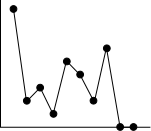[Home]

# Probability Theory I

This is the class webpage for Probablity Theory I (2018).
• Teacher: Arnab Chakraborty
• Office: Room 914, ASU, 9-th floor of S N Bose Bhavan.
• Email:arnabc at isical dot ac in
Alternatively, you may use this diagram to contact me:If you want to leave an anonymous note for me, just use the comment box provided at the bottom of each page.

## Class notes

All the lecture notes are available from the class notes page. These notes are the definitive source of material for this course. The practice problems given in the notes are indicative of the problems you are likely to face in the exams.

## Survival tips

Be sure to read the survival tips.

## Textbooks

We shall not follow any single textbook. All the material that we shall cover may be found in the following books.
• A First Course in Probability Theory by Sheldon Ross: The book is easy to read. We shall cover only about half the book in this semester. Cheap Indian edition available.
• Probability Theory (Vol 1) by William Feller: A classic book which (in my opinion) is not very well-organised. But it contains lots of discussions on practical applications of probability. We shall pick some advanced topics from this book from time to time. Cheap Indian edition available. Most students buy one copy of this book, and then never read it.
• Counterexamples in Probability by Jordan M Stoyanov: Lots of fun examples to baffle (and improve) your intuition. We shall borrow some of these examples.
• Fifty challenging problem in probability with solutions by Frederick Mosteller

## Syllabus

Up to midsem: Elementary concepts: experiments, outcomes, sample space, events. Discrete sample spaces and probability models. Equally likely set-up and combinatorial probability. Fluctuations in coin tossing and random walks, Combination of events. Composite experiments, conditional probability, Polya's urn scheme, Bayes theorem, independence. Discrete random variables. Expectation/mean, functions of discrete random variables,

After midsem: Variance, moments, moment generating functions probability generating functions. Standard discrete distributions. Joint distributions of discrete random variables, independence, conditional distributions, conditional expectation. Distribution of sum of two independent random variables. Functions of more than one discrete random variables.